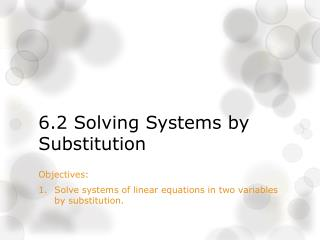DownloadDownload Presentation6.2 Solving Systems by Substitution

# 6.2 Solving Systems by Substitution

Télécharger la présentation## 6.2 Solving Systems by Substitution

- - - - - - - - - - - - - - - - - - - - - - - - - - - E N D - - - - - - - - - - - - - - - - - - - - - - - - - - -
##### Presentation Transcript

1. 6.2 Solving Systems by Substitution Objectives: Solve systems of linear equations in two variables by substitution.

2. Example 1: Solve for x when – A. B. C. D.

3. Why is graphing not always the most useful way to solve a system of equations? • We don’t always land on whole numbers! • Remember – when solving a system of linear equations we are looking for an ordered pair that makes both equations true. • We will use substitution when: • Both equations are solved for 1 variable. • 1 equation is solved for a variable, and one is in standard form.

4. Example 2: continued... C. D.

5. Homework • Page 347: 1, 2, 8-11

6. Example 3: Josie is deciding between two cell phone plans. The first plan has a \$50 sign-up fee and costs \$20 a month. The second plan has a \$30 sign-up fee and costs \$25 a month. • After how many months will the total cost be the same? • What will the cost be? • If Josie has to sign up for a one year contract, which plan would be cheaper?

7. Trivia 1. Which TV show has the judges: Mariah Carey, Nicki Minaj, Randy Jackson, & Keith Urban? American Idol 2. Which Disney movie has the characters: Prince Eric, Sebastian, Flounder, Ursula, and King Triton? Little Mermaid

8. If both equations are in standard form: You must solve one of them for a variable and then substitute.

9. Example 4: Solve each system by substitution. A. B.

10. Practice: • Practice 6.2 WS

11. Homework • Page 347: 8-23 odd